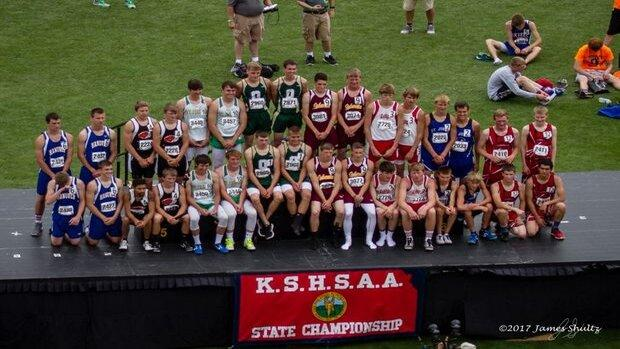# PreSeason Class 3A Team Rankings

##These rankings were calculated by scoring the rankings as a meet. We took the individual rankings and relay rankings and scored them as a normal meet: 10-8-6-5-4-3-2-1.

Athletes whose graduation years are unknown were left out of the list.

Relay teams are also not included in preseason rankings

## Boys

1.

### 41

Brennan Walker (J 8 = 8)
Bryce Mason (J 4 + S 1 = 5)
Creighton Johnson (D 8 + S 5 = 13)
Vincent Palen (110H 10 + 300H 3 + TJ 2 = 15)
2.

### 36.5

Brandon Winderlin (400m 6 = 6)
Hunter Yager (PV 5.5 = 5.5)
Kaden Wren (400m 8 + 800m 8 = 16)
Loren Faurot (PV 9 = 9)
3.

### 29

Isaiah Armstrong (100m 3 + 200m 10 + 400m 10 = 23)
Zephyn Mason (LJ 6 = 6)
4.

### 28.43

Gideon Remer (D 6 = 6)
Kyler Kaniper (1600m 4 + 3200m 3 + HJ 1.43 = 8.43)
Logan Matzke (300H 6 + TJ 8 = 14)
5.

### 28

Chase Poague (110H 2 = 2)
Dominic Jackson (3200m 1 = 1)
Dylan Sprecker (1600m 5 + 3200m 4 = 9)
Luke Gleason (1600m 10 + 3200m 6 = 16)
6.

### 25.33

Braden Black (J 3 = 3)
Elijah Judd (200m 4 = 4)
Riley Petz (110H 8 + 300H 10 = 18)
Seth Teague (PV 0.33 = 0.33)
7.

### 21.5

Brett Schwartz (D 10 = 10)
Draven Schallock (200m 6 = 6)
Evan Dean (S 3 = 3)
Ryan Weekly (200m 2.5 = 2.5)
7.

### 21.5

Carter Jones (PV 5.5 = 5.5)
Connor Hall (1600m 8 + 3200m 8 = 16)
9.

### 20

Gavin Cumpton (110H 6 + 300H 4 = 10)
Kain Fink (HJ 10 = 10)
9.

### 20

Gabe Valentine (100m 10 + LJ 10 = 20)